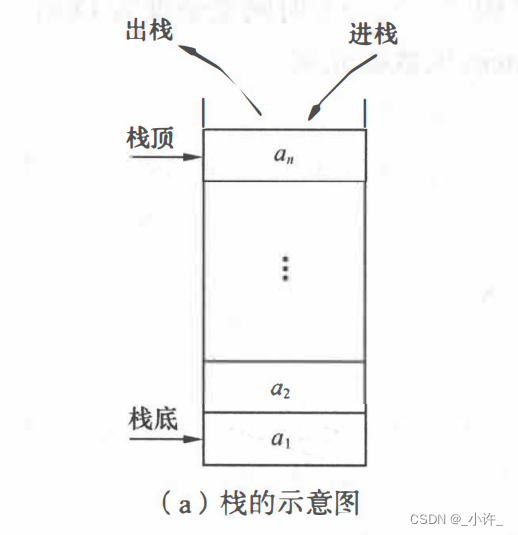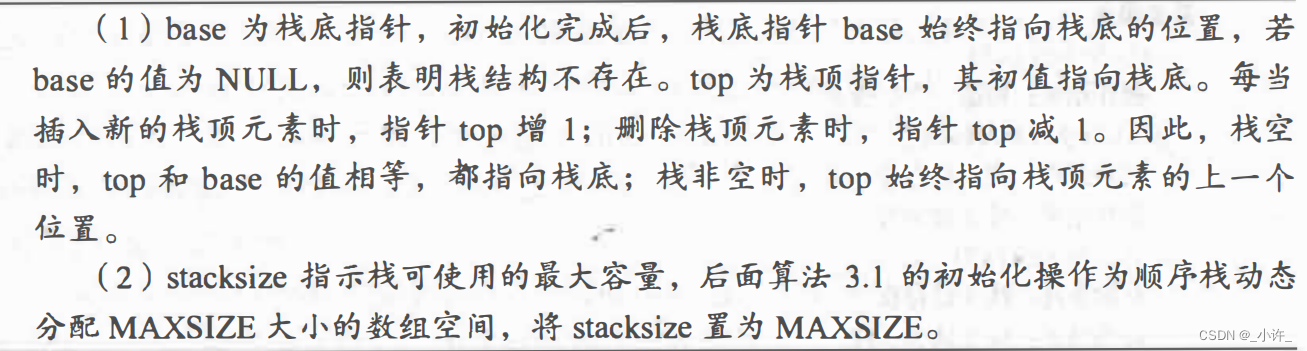# 顺序栈与链栈

### 简介### 顺序栈• 序列栈
#include<stdio.h>
#include<stdlib.h>
#define MAXSIZE 100

typedef struct{

int *base;      //栈顶指针
int *top;      //栈底指针
int StackSize;
}SqStack;

//初始化
SqStack InitStack(){

SqStack S;
int a [MAXSIZE];
S.base =  a;
S.top = S.base;
S.StackSize = MAXSIZE;
return S;
}

//入栈
int Push(SqStack *S, int e){

if(S->top-S->base ==S->StackSize) return 0;   //栈满
S->top = e;
(*S).top++;             //S->top ++
return 1;
}

//出栈
int Pop(SqStack *S){

if(S->base == S->top) return 0;    //栈空
(*S).top--;              //S->top--
int e;
e= S->top;
return e;
}

int main(){

SqStack  S = InitStack();
Push(&S,1);
int e = Pop(&S);
printf("%d\n",e);
Push(&S,2);
int e1 = Pop(&S);
printf("%d\n",e1);
Push(&S,3);
int e3 = Pop(&S);
printf("%d\n",e3);
return 0;

}


### 链栈

#include<stdio.h>
#include<stdlib.h>

typedef struct StackNode{

int data;
struct StackNode *next;

L->next = NULL;
return L;
}

//入栈-----栈的特点显示链栈只能使用前插法

StackNode* node = (StackNode*)malloc(sizeof(StackNode));
node->data = e;
node->next = L->next;
L->next = node;
return 1;
}

//出栈 (后进先出)

if(L->next ==NULL) return 0;
int e;
e= L->next->data;
L->next=L->next->next;
return e;
}

int main(){

Push(L,2);
Push(L,3);
int e = Pop(L);
printf("%d",e);
int e1 = Pop(L);
printf("%d",e1);
return 0;
}


### 栈与递归

1. 子问题必须与原问题相同，但更简单；

2. 它不能无限制地调用自身，必须有出口，并且可以简化为非递归情况处理。

int Fn(int x){

if(x==1) return 1;
else return Fn(x-1)+x;
}

#include<stdio.h>

int Fn(int x){

if(x==1) return 1;
else return Fn(x-1)+x;
}

int main(){

int e = Fn(5);
printf("%d",e);
}

. . .

## 相关推荐

#### 【Python】【Selenium】如何给Input框输入带格式内容

【背景】 用selenium写自动化脚本过程中涉及向输入框自动传输内容。而且有时内容很多，如何保持段落分行呢？ 【代码】 resource_desc = driver.find_element_by_xpath("//textarea[contains(

#### Python 第九节 第三课

[toc] try...多个 except 结构     上面的结构可以捕获所有的异常, 工作中也很常见. 但是, 从金典理论考虑, 一般建议尽量捕获可能出现的多个异常 ( 按照先子类后父类的顺序 ) , 并且针对性的写出异常处理多代码, 为了避免遗漏可

28天前
28天前
27天前
27天前
25天前
25天前
23天前
17天前

28天前
28天前
27天前
25天前
25天前
25天前
25天前
24天前
24天前
21天前